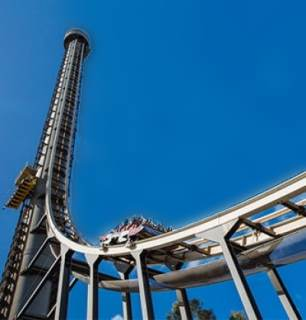# Activities

•• ##### Subject Area

• Mathematics: Algebra and Pattern: Algebra and Pattern
• Mathematics: Calculus: Calculus
• Mathematics: Statistics and Data Analysis: Statistics and Data Analysis

• ##### AuthorAust Senior

90 Minutes

• ##### Device
• TI-Nspire™ CAS
• ##### Software

TI-Nspire™ CAS

3.2

• ##### Other Materials
Dynamic wireless data device + data-vest, Lab quest 2 with G.P.S capabilities

## Amusement Park Rides#### Activity Overview

Year 11 students from St Ursula’s College spent the day at Dreamworld collecting data for their assignments which were to be completed the following term. Data devices such as the wireless dynamic sensor and iPhones were used to collect data which was analysed with TI-Nspire calculators and software. Other data collection included the use of clinometers to measure angles, mobile phones for timing and video recording.

#### Objectives

• Determine quartiles and inter-quartile range
• Solve problems involving direct proportion.
• Explore the relationship between the graphs and equations corresponding to simple rate problems.
• Explore the connection between algebraic and graphical representations of relations such as simple quadratics, circles and exponentials using digital technology as appropriate

#### Vocabulary

• Altitude
• Velocity
• Box-plot
• Derivative
• Periodic motion
• Pendulum
• Regression Analysis
• Terminal Velocity